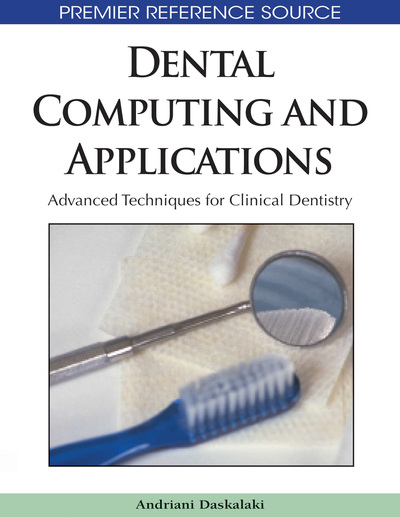# 3D Reconstructions from Few Projections in Oral Radiology

Ralf K.W. Schulze
DOI: 10.4018/978-1-60566-292-3.ch007
OnDemand:
(Individual Chapters)
Available
\$37.50
No Current Special Offers

## Abstract

Established techniques for three-dimensional radiographic reconstruction such as computed tomography (CT) or, more recently cone beam computed tomography (CBCT) require an extensive set of measurements/ projections from all around an object under study. The x-ray dose for the patient is rather high. Cutting down the number of projections drastically yields a mathematically challenging reconstruction problem. Few-view 3D reconstruction techniques commonly known as “tomosynthetic reconstructions” have gained increasing interest with recent advances in detector and information technology.
Chapter Preview
Top

## Radiographic Projection And 3D Reconstruction From Multiple Projections

The projection value measured by any x-ray sensitive receptor follows the well-known Lambert-Beer law:

(1) with I1 defining the intensity behind an absorber and I0 the input intensity, respectively. The parameters μ and d denote the mass absorption coefficient and the thickness of the absorber. We aim to estimate μ as shade of gray at discrete instances to obtain a reliable representation of the object.

In 1917, the Austrian mathematician Johann Radon discovered that the two-dimensional (2D) distribution of properties of an object may be obtained from an infinite number of line integrals sampled through the object. Mathematically, a functioncan be completely described by the complete number of straght line integrals through the support of, i.e.

(2)

The famous method predominantly applied for image reconstructions in CT-scanners uses this formula in a process termed “Filtered Backprojection (FBP)”. Assuming parallel x-rays, the relationship between the projection data (P) and the object are given by:

(3) where Θ denotes the projection angle and t the detector position in the beam (Fig. 1). Diracs delta function δ is required to define the line interval. The CT image reconstruction problem is to computegiven. Note, that the term “” in equation (3)” represents a line equation (e.g. green “ray-line” in Fig. 1), (Beyerer & Leon, 2002) the sum of which the integrals are sampled. In other words, the measured data on the image receptor represent the integrals over a finite number of lines connecting the x-ray source with the detector cells.

## Complete Chapter List

Search this Book:
Reset